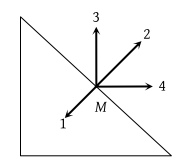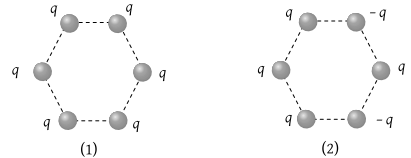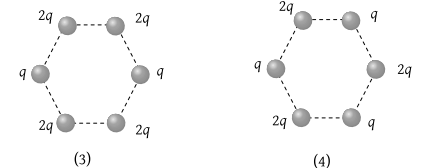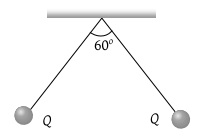Three identical point charges, as shown are placed at the vertices of an isosceles right angled triangle. Which of the numbered vectors coincides in direction with the electric field at the mid-point M of the hypotenuse(1) 1

(2) 2

(3) 3

(4) 4

High Yielding Test Series + Question Bank - NEET 2020

Difficulty Level:

Figures below show regular hexagons, with charges at the vertices. In which of the following cases the electric field at the centre is not zero?(1) 1

(2) 2

(3) 3

(4) 4

Concept Questions :-

Electric field
High Yielding Test Series + Question Bank - NEET 2020

Difficulty Level:

An electron enters an electric field with its velocity in the direction of the electric lines of force. Then

(1) The path of the electron will be a circle

(2) The path of the electron will be a parabola

(3) The velocity of the electron will decrease

(4) The velocity of the electron will increase

Concept Questions :-

Electric field
High Yielding Test Series + Question Bank - NEET 2020

Difficulty Level:

The dimension of (1/2) : permittivity of free space; E: electric field) is

(1) MLT–1

(2) ML2L–2

(3) ML–1T–2

(4) ML2T–1

Concept Questions :-

Electric field
High Yielding Test Series + Question Bank - NEET 2020

Difficulty Level:

Two small spherical balls each carrying a charge Q = 10 μC (10 micro-coulomb) are suspended by two insulating threads of equal lengths 1m each, from a point fixed in the ceiling. It is found that in equilibrium threads are separated by an angle 60° between them, as shown in the figure. What is the tension in the threads (Given: $\frac{1}{\left(4\pi {\epsilon }_{0}\right)}=9×{10}^{9}Nm/{C}^{2}$)(1) 18 N

(2) 1.8 N

(3) 0.18 N

(4) None of the above

Concept Questions :-

Coulomb's law
High Yielding Test Series + Question Bank - NEET 2020

Difficulty Level:

An electron having charge ‘e’ and mass ‘m’ is moving in a uniform electric field E. Its acceleration will be

(1) $\frac{{e}^{2}}{m}$

(2) $\frac{{E}^{2}e}{m}$

(3) $\frac{eE}{m}$

(4) $\frac{mE}{e}$

Concept Questions :-

Electric field
High Yielding Test Series + Question Bank - NEET 2020

Difficulty Level:

A table tennis ball that has been covered with conducting paint is suspended by a silk thread so that it hangs between two plates, out of which one is earthed and other is connected to a high voltage generator. This ball

(1) Is attracted towards high voltage plate and stays there

(2) Hangs without moving

(3) Swing backward and forward hitting each plate in turn

(4) Is attracted to the earthed plate and stays there

Concept Questions :-

Ways of charging
High Yielding Test Series + Question Bank - NEET 2020

Difficulty Level:

If an insulated non-conducting sphere of radius R has charge density ρ. The electric field at a distance r from the centre of sphere (r < R) will be

(1) $\frac{\rho \text{\hspace{0.17em}}R}{3{\epsilon }_{0}}$

(2) $\frac{\rho \text{\hspace{0.17em}}r}{{\epsilon }_{0}}$

(3) $\frac{\rho \text{\hspace{0.17em}}r}{3{\epsilon }_{0}}$

(4) $\frac{3\rho \text{\hspace{0.17em}}R}{{\epsilon }_{0}}$

Concept Questions :-

Electric field
High Yielding Test Series + Question Bank - NEET 2020

Difficulty Level:

Infinite charges of magnitude q each are lying at x =1, 2, 4, 8... meter on X-axis. The value of the intensity of the electric field at point x = 0 due to these charges will be

(1) 12 × 109q N/C

(2) Zero

(3) 6 × 109q N/C

(4) 4 × 109q N/C

Concept Questions :-

Electric field
High Yielding Test Series + Question Bank - NEET 2020

Difficulty Level:

A pendulum bob of mass $30.7×{10}^{-6}\text{\hspace{0.17em}}kg$ and carrying a charge $2×{10}^{-8}\text{\hspace{0.17em}}C$ is at rest in a horizontal uniform electric field of 20000 V/m. The tension in the thread of the pendulum is $\left(g=9.8\text{\hspace{0.17em}}m/{s}^{2}\right)$

(1) $3×{10}^{-4}\text{\hspace{0.17em}}N$

(2) $4×{10}^{-4}\text{\hspace{0.17em}}N$

(3) $5×{10}^{-4}\text{\hspace{0.17em}}N$

(4) $6×{10}^{-4}\text{\hspace{0.17em}}N$

Concept Questions :-

Electric field